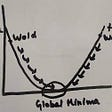# Elementary Neural Networks with TensorFlow

I recently had a chance to look into TensorFlow, Google’s “open source software library for numerical computation using data flow graphs,” specifically looking to implement artificial neural networks (ANNs). Most of the introductory tutorials on ANNs with TensorFlow involve building and training networks to classify handwritten digits. In order to better learn the building blocks of TensorFlow—and to refresh my memory of both Python and neural networks—I wanted to start much much smaller, beginning with the simplest possible ANNs and working my way up.

I’ll describe building and training perceptrons to perform boolean operations using TensorFlow’s Python API. In order to focus on implementation for newcomers (like myself) to TensorFlow, I’ll assume basic background knowledge concerning Python and ANNs.

## AND

First, we’ll build and train a single-layer perceptron, with a constant bias input and a step activation function, to perform the AND operation. Here is a diagram of the model we’re going to build:

First, import the TensorFlow package:

`import tensorflow as tf`

Our training data consists of the truth table for AND, with the four possible pairs of operands as our inputs, and the operation’s respective results as our outputs. Note that the bias is implemented by adding an extra value of 1 to all training examples.

`T, F = 1., -1.bias = 1.train_in = [    [T, T, bias],    [T, F, bias],    [F, T, bias],    [F, F, bias],]train_out = [    [T],    [F],    [F],    [F],]`

TensorFlow works by building a model out of empty tensors, then plugging in known values and evaluating the model. Since the above training data will remain constant, the only special TensorFlow object we have to worry about in this case is our 3 x 1 tensor of weights:

`w = tf.Variable(tf.random_normal([3, 1]))`

That’s a tensor that is variable — so its value may be changed on each evaluation of the model as we train—with all values initialized to normally-distributed random numbers.

Now that we have our training data and weight tensor, we have everything needed to build our model using TensorFlow’s comprehensive library of functions. Many useful activation functions are included, but we’ll write our own simple step function with a threshold of 0:

`# step(x) = { 1 if x > 0; -1 otherwise }def step(x):    is_greater = tf.greater(x, 0)    as_float = tf.to_float(is_greater)    doubled = tf.mul(as_float, 2)    return tf.sub(doubled, 1)`

With the step function defined, the output, error, and mean squared error of our model can be calculated in one short line each:

`output = step(tf.matmul(train_in, w))error = tf.sub(train_out, output)mse = tf.reduce_mean(tf.square(error))`

The evaluation of certain tensor functions can also update the value of variables, like our tensor of weights w. First we calculate the desired adjustment based on error, then add it to w:

`delta = tf.matmul(train_in, error, transpose_a=True)train = tf.assign(w, tf.add(w, delta))`

The model has to be evaluated by a TensorFlow session, which we instantiate before initializing all variables to their specified values:

`sess = tf.Session()sess.run(tf.initialize_all_variables())`

We can now run our model through training epochs, adjusting the weights each time by evaluating train. Since we’re using a binary output, we can expect to reach a perfect result with a mean squared error of 0. We will reach it very quickly, but it’s a good idea to set a maximum number of epochs to be sure there won’t be an infinite loop:

`err, target = 1, 0epoch, max_epochs = 0, 10while err > target and epoch < max_epochs:    epoch += 1    err, _ = sess.run([mse, train])    print('epoch:', epoch, 'mse:', err)`

Note that Session.run will return the result of whatever is evaluated. On each epoch, we evaluate mse in order to track progress, and train to actually adjust the weights.

If you go ahead and run the code, this extremely simple network should arrive at a solution in just a few training epochs.

## OR

Training this network to perform OR is an equivalent problem. Just change the desired output (train_out) to appropriate truth values and the network will arrive at a solution just as quickly.

## XOR

XOR is a very different problem, and will require a slightly more complex model. It will change in the following ways:

• a hidden layer of two nodes will be added
• instead of a constant bias, variable biases will be added to each weight
• hyperbolic tangent activation functions will be used
`import tensorflow as tfT, F = 1., -1.train_in = [ [T, T], [T, F], [F, T], [F, F],]train_out = [ [F], [T], [T], [F],]w1 = tf.Variable(tf.random_normal([2, 2]))b1 = tf.Variable(tf.zeros())w2 = tf.Variable(tf.random_normal([2, 1]))b2 = tf.Variable(tf.zeros())out1 = tf.tanh(tf.add(tf.matmul(train_in, w1), b1))out2 = tf.tanh(tf.add(tf.matmul(out1, w2), b2))error = tf.sub(train_out, out2)mse = tf.reduce_mean(tf.square(error))`

Training this multilayer network involves a much more complex gradient descent algorithm, which TensorFlow will handle for us. Here we set it to minimize mean squared error with a learning rate of 0.01:

`train = tf.train.GradientDescentOptimizer(0.01).minimize(mse)`

Now we run our training epochs just as before. Since we’re not dealing with binary outputs anymore, we wouldn’t expect to reach an error of o. This training will also require many more epochs to converge on a reasonable solution:

`sess = tf.Session()sess.run(tf.initialize_all_variables())err, target = 1, 0.01epoch, max_epochs = 0, 5000while err > target and epoch < max_epochs:   epoch += 1   err, _ = sess.run([mse, train])print(‘epoch:’, epoch, ‘mse:’, err)`

## Conclusion

Hopefully this overview has provided a digestible introduction to TensorFlow that will allow you to dive into more realistic applications.

For a more in-depth implementation and discussion of training an XOR network, see Stephen Oman’s excellent article Solving XOR with a Neural Network in TensorFlow; or dive right in to TensorFlow’s tutorials.

--

--

--

## More from Jascha Ephraim

Love podcasts or audiobooks? Learn on the go with our new app.## All you have to know about Multi-Layer Neural Networks!## Kaggle Intel Image Classification (~94% accuracy)— Project## 3 ways to create a Deep Neural Network model with Keras and Tensorflow 2.0## Hand Segmentation with Python and Tensorflow